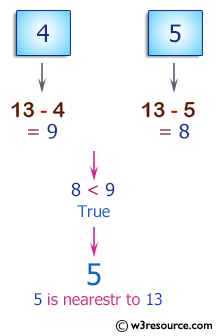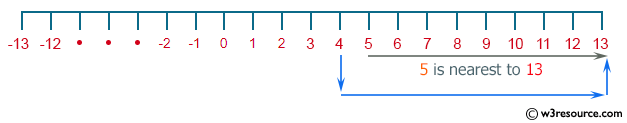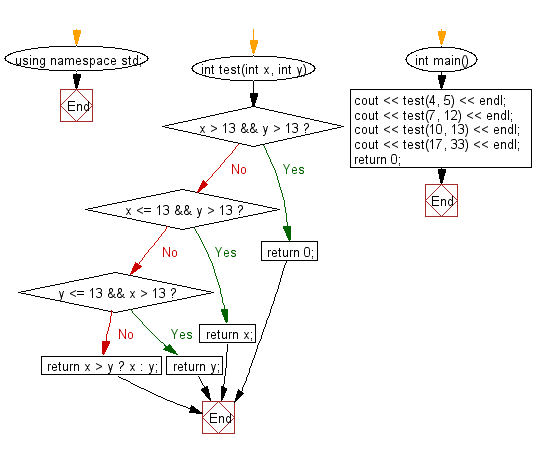﻿ C++ : Check two integers, return whichever is nearest to 13# C++ Exercises: Check two given integers and return the value whichever value is nearest to 13 without going over

## C++ Basic Algorithm: Exercise-55 with Solution

Write a C++ program to check two given integers and return the value whichever value is nearest to 13 without going over. Return 0 if both numbers go over.

Sample Solution:

C++ Code :

``````#include <iostream>
using namespace std;

int test(int x, int y)
{
if (x > 13 && y > 13) return 0;
if (x <= 13 && y > 13) return x;
if (y <= 13 && x > 13) return y;
return x > y ? x : y;
}

int main()
{
cout << test(4, 5) << endl;
cout << test(7, 12) << endl;
cout << test(10, 13) << endl;
cout << test(17, 33) << endl;
return 0;
}
``````

Sample Output:

```5
12
13
0
```

Pictorial Presentation:Flowchart:C++ Code Editor: